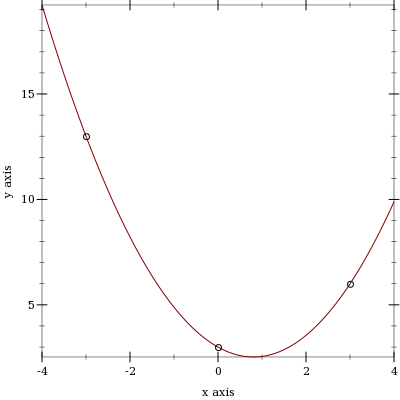#### 7.3Construction

syntax

(matrix [[expr ...+] ...+] maybe-type-ann)

maybe-type-ann =
| : type
Like the array form for creating arrays, but does not require #[...] to delimit nested rows, and the result is constrained to be a matrix?.

Examples:

 > (matrix [[1 2 3] [4 5 6]]) - : (Array Positive-Byte) (array #[#[1 2 3] #[4 5 6]]) > (matrix [[1 2 3] [4 5 6]] : Number) - : (Array Number) (array #[#[1 2 3] #[4 5 6]]) > (matrix [[]]) eval:20:0: matrix: given empty row at: () in: (matrix (()))

syntax

(row-matrix [expr ...+] maybe-type-ann)

maybe-type-ann =
| : type
Like matrix, but returns a row matrix.

Examples:

 > (row-matrix [1 2 3]) - : (Array Positive-Byte) (array #[#[1 2 3]]) > (row-matrix [1 2 3] : Number) - : (Array Number) (array #[#[1 2 3]]) > (row-matrix []) eval:23:0: row-matrix: given empty row at: () in: (row-matrix ())

syntax

(col-matrix [expr ...+] maybe-type-ann)

maybe-type-ann =
| : type
Like matrix, but returns a column matrix.

Examples:

 > (col-matrix [1 2 3]) - : (Array Positive-Byte) (array #[# # #]) > (col-matrix [1 2 3] : Number) - : (Array Number) (array #[# # #]) > (col-matrix []) eval:26:0: col-matrix: given empty column at: () in: (col-matrix ())

 procedure(identity-matrix n [one zero]) → (Matrix A) n : Integer one : A = 1 zero : A = 0
Returns an n×n identity matrix, which has the value one on the diagonal and zero everywhere else. The height/width n must be positive.

Examples:

> (identity-matrix 3)

- : (Array (U Zero One))

(array #[#[1 0 0] #[0 1 0] #[0 0 1]])

> (identity-matrix 4 1.0+0.0i 0.0+0.0i)

- : (Array Float-Complex)

 (array #[#[1.0+0.0i 0.0+0.0i 0.0+0.0i 0.0+0.0i] #[0.0+0.0i 1.0+0.0i 0.0+0.0i 0.0+0.0i] #[0.0+0.0i 0.0+0.0i 1.0+0.0i 0.0+0.0i] #[0.0+0.0i 0.0+0.0i 0.0+0.0i 1.0+0.0i]])

 procedure(make-matrix m n x) → (Matrix A) m : Integer n : Integer x : A
Returns an m×n matrix filled with the value x; both m and n must be positive. Analogous to make-array (and defined in terms of it).

 procedure(build-matrix m n proc) → (Matrix A) m : Integer n : Integer proc : (Index Index -> A)
Returns an m×n matrix with entries returned by proc; both m and n must be positive. Analogous to build-array (and defined in terms of it).

 procedure(diagonal-matrix xs [zero]) → (Matrix A) xs : (Listof A) zero : A = 0
Returns a matrix with xs along the diagonal and zero everywhere else. The length of xs must be positive.

Examples:

> (diagonal-matrix '(1 2 3 4 5 6))

- : (Array Byte)

 (array #[#[1 0 0 0 0 0] #[0 2 0 0 0 0] #[0 0 3 0 0 0] #[0 0 0 4 0 0] #[0 0 0 0 5 0] #[0 0 0 0 0 6]])
> (diagonal-matrix '(1.0 2.0 3.0 4.0 5.0) 0.0)

- : (Array (U Positive-Float Flonum-Positive-Zero))

 (array #[#[1.0 0.0 0.0 0.0 0.0] #[0.0 2.0 0.0 0.0 0.0] #[0.0 0.0 3.0 0.0 0.0] #[0.0 0.0 0.0 4.0 0.0] #[0.0 0.0 0.0 0.0 5.0]])

 procedure(block-diagonal-matrix Xs [zero]) → (Matrix A) Xs : (Listof (Matrix A)) zero : A = 0
Returns a matrix with matrices Xs along the diagonal and zero everywhere else. The length of Xs must be positive.

Examples:

 > (block-diagonal-matrix (list (matrix [[6 7] [8 9]]) (diagonal-matrix '(7 5 7)) (col-matrix [1 2 3]) (row-matrix [4 5 6])))

- : (Array Byte)

 (array #[#[6 7 0 0 0 0 0 0 0] #[8 9 0 0 0 0 0 0 0] #[0 0 7 0 0 0 0 0 0] #[0 0 0 5 0 0 0 0 0] #[0 0 0 0 7 0 0 0 0] #[0 0 0 0 0 1 0 0 0] #[0 0 0 0 0 2 0 0 0] #[0 0 0 0 0 3 0 0 0] #[0 0 0 0 0 0 4 5 6]])
 > (block-diagonal-matrix (list (make-matrix 2 2 2.0+3.0i) (make-matrix 2 2 5.0+7.0i)) 0.0+0.0i)

- : (Array Float-Complex)

 (array #[#[2.0+3.0i 2.0+3.0i 0.0+0.0i 0.0+0.0i] #[2.0+3.0i 2.0+3.0i 0.0+0.0i 0.0+0.0i] #[0.0+0.0i 0.0+0.0i 5.0+7.0i 5.0+7.0i] #[0.0+0.0i 0.0+0.0i 5.0+7.0i 5.0+7.0i]])

 procedure(vandermonde-matrix xs n) → (Matrix Number) xs : (Listof Number) n : Integer
Returns an m×n Vandermonde matrix, where m = (length xs).

Examples:

> (vandermonde-matrix '(1 2 3 4) 5)

- : (Array Real)

(array #[#[1 1 1 1 1] #[1 2 4 8 16] #[1 3 9 27 81] #[1 4 16 64 256]])

> (vandermonde-matrix '(5.2 3.4 2.0) 3)

- : (Array Flonum)

 (array #[#[1.0 5.2 27.040000000000003] #[1.0 3.4 11.559999999999999] #[1.0 2.0 4.0]])
Using a Vandermonde matrix to find a Lagrange polynomial (the polynomial of least degree that passes through a given set of points):
 > (define (lagrange-polynomial xs ys) (array->list (matrix-solve (vandermonde-matrix xs (length xs)) (->col-matrix ys))))
> (define xs '(-3 0 3))
> (define ys '(13 3 6))
> (match-define (list c b a) (lagrange-polynomial xs ys))
 > (plot (list (function (λ (x) (+ c (* b x) (* a x x))) -4 4) (points (map list xs ys))))Note that the above example is in untyped Racket.

This function is defined in terms of array-axis-expand.

syntax

 (for/matrix: m n maybe-fill (for:-clause ...) maybe-type-ann body ...+)

syntax

 (for*/matrix: m n maybe-fill (for:-clause ...) maybe-type-ann body ...+)

maybe-fill =
| #:fill fill

maybe-type-ann =
| : body-type

 m : Integer
 n : Integer
 fill : body-type
Like for/array: and for*/array:, but for matrices. The only material difference is that the shape m n is required and must be positive.

syntax

 (for/matrix m n maybe-fill (for-clause ...) body ...+)

syntax

 (for*/matrix m n maybe-fill (for-clause ...) body ...+)
Untyped versions of the loop macros.